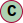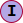# Class Pose

Position and orientation.

In computer vision and robotics, a typical task is to identify specific objects in an image and to determine each object's position and orientation relative to some coordinate system. This information can then be used, for example, to allow a robot to manipulate an object or to avoid moving into the object. The combination of position and orientation is referred to as the pose of an object, even though this concept is sometimes used only to describe the orientation. Exterior orientation and translation are also used as synonyms of pose.

### See

https://en.wikipedia.org/wiki/Pose_(computer_vision)

#### Hierarchy-Diagram##### Legendclassinterface

## Properties

elements: number[]
timestamp: number = ...

Position recording timestamp

unit: LengthUnit = LengthUnit.METER

Position unit

## Accessors

• get probability(): number
• Get the position probability

### Returns

Probability between 0 and 1

#### Returns number

• set probability(value: number): void
• Position probability

## Methods

• Sets this matrix to the transformation composed of translation, rotation and scale.

• ### Deprecated

#### Returns void

• Decomposes this matrix into it's position, quaternion and scale components.

#### Returns Matrix4

• Check if this position equals another position

#### Parameters

• ##### matrix: Matrix4

Other position

• ### Deprecated

#### Returns Matrix4

• Copies the rotation component of the supplied matrix m into this matrix rotation component.

• ### Deprecated

#### Returns number[]

• Sets the values of this matrix from the provided array or array-like.

#### Parameters

• ##### array: number[] | ArrayLike<number>

the source array or array-like.

• ##### `Optional` offset: number

(optional) offset into the array-like. Default is 0.

• ### Deprecated

• #### Returns number

• Resets this matrix to identity.

#### Returns Matrix4

• Inverts this matrix.

#### Returns Matrix4

• Constructs a rotation matrix, looking from eye towards center with defined up vector.

#### Returns Matrix4

• Creates an orthographic projection matrix.

#### Returns Matrix4

• Creates a frustum matrix.

#### Returns Matrix4

• Creates a perspective projection matrix.

#### Returns Matrix4

• Sets this matrix as rotation transform around axis by angle radians. Based on http://www.gamedev.net/reference/articles/article1199.asp.

#### Parameters

• ##### axis: Vector3

Rotation axis.

#### Returns Matrix4

• Sets this matrix as rotation transform around x axis by theta radians.

#### Returns Matrix4

• Sets this matrix as rotation transform around y axis by theta radians.

#### Returns Matrix4

• Sets this matrix as rotation transform around z axis by theta radians.

#### Returns Matrix4

• Sets this matrix as scale transform.

#### Returns Matrix4

• Sets this matrix as shear transform.

#### Returns Matrix4

• Sets this matrix as translation transform.

#### Returns Matrix4

• Multiplies this matrix by m.

#### Returns Matrix4

• Sets this matrix to a x b.

#### Returns Matrix4

• Multiplies this matrix by s.

#### Returns Matrix4

• Sets this matrix to a x b and stores the result into the flat array r. r can be either a regular Array or a TypedArray.

### Deprecated

This method has been removed completely.

• ### Deprecated

This method has been removed completely.

• ### Deprecated

#### Returns void

• Multiplies the columns of this matrix by vector v.

#### Returns Matrix4

• Sets all fields of this matrix.

#### Returns Matrix4

• Set the upper 3x3 elements of this matrix to the values of the Matrix3 m.

#### Returns Matrix4

• Sets the position component for this matrix from vector v.

• ### Deprecated

#### Returns Matrix4

• Returns an array with the values of this matrix, or copies them into the provided array.

### Returns

The created or provided array.

#### Parameters

• ##### `Optional` array: number[]

(optional) array to store the matrix to. If this is not provided, a new array will be created.

• ##### `Optional` offset: number

(optional) optional offset into the array.

#### Returns Matrix4Tuple

• Copies he values of this matrix into the provided array-like.

### Returns

The provided array-like.

#### Parameters

• ##### `Optional` array: ArrayLike<number>

array-like to store the matrix to.

• ##### `Optional` offset: number

(optional) optional offset into the array-like.

#### Returns ArrayLike<number>

• Transposes this matrix.

#### Returns Matrix4

• Create a matrix from array

### Returns

Matrix4

Array

#### Returns T

• Get a pose from a 4d matrix

### Returns

Pose instance

4x4 Matrix

#### Returns T

• Create a pose from a position

### Returns

Output pose

#### Parameters

• ##### position: Absolute3DPosition

3D position

#### Returns T

• Create a rotation matrix from euler angles

### Returns

Rotation matrix

Vector

Angle

#### Returns T

• Create a rotation matrix from euler angles

### Returns

Rotation matrix

#### Parameters

• ##### euler: Euler

Euler angles

#### Returns T

• Create a rotation matrix from quaternion

### Returns

Rotation matrix

Quaternion